# RBSE Maths Class 9 Chapter 11: Important Questions and Solutions

RBSE Maths Chapter 11 – Area of Plane Figures Class 9 Important questions and solutions are provided here. The important questions and solutions of Chapter 11 are available at BYJU’S given by the expert faculty, along with detailed explanations for each and every question. Also, RBSE Class 9 solutions are available so that students can refer to them to excel their skills.

Chapter 11 Area of plane figures of the RBSE Class 9 Maths contains different concepts on areas of various types of plane figures. After getting through with all these important questions, students will be able to solve the questions of finding area, perimeter, missing sides, diagonals and so on. These concepts will help the students in their higher classes also.

## RBSE Maths Chapter 11: Exercise 11.1 Textbook Important Questions and Solutions

Question 1: If the base of a triangle is 20 cm and its height is 6 cm, then find the area of the triangle.

Solution:

Given,

Base = 20 cm

Height = 6 cm

Area of triangle = ½ × base × height

= ½ × 20 × 6

= 60 cm2

Question 2: A triangle whose sides are 15 cm, 25 cm and 30 cm, respectively, then find the area of the triangle.

Solution:

Let a, b and c be the sides of the triangle.

a = 15 cm, b = 25 cm and c = 30 cm

Semi-perimeter of triangle:

s = (a + b + c)/2

= (15 + 25 + 30)/2

= 70/2

= 35

Area of triangle = √[s(s – a)(s – b)(s – c)]

= √[35 (35 – 15)(35 – 25)(35 – 30)]

= √(35 × 20 × 10 × 5)

=√( 7 × 5 × 5 × 4 × 2 × 5 × 5)

= 5 × 5 × 2 √(7 × 2)

= 50√14 cm2

Question 3: In an isosceles triangle each equal side is 8 cm and the third side is 4 cm, then find its area.

Solution:

Let a = 8 cm and b = 4 cm

Area of an isosceles triangle = (b/4) × √(4a2 – b2)

= (4/4) × √[4(8)2 – 42]

= √[4(64) – 16]

= √(256 – 16)

= √240

= 4√15 cm2

Question 4: An equilateral triangle whose side is 20 cm, then find its area.

Solution:

Given,

Side of the triangle = a = 20 cm

Area of triangle = (√3)/4 a2

= (√3)/4 × (20)2

= (√3)/4 × 400

= 100√3 cm2

Question 5: A triangle whose sides are 8 cm and 15 cm and its perimeter is 40 cm then find the area of the triangle.

Solution:

Let a, b and c be the sides of the triangle.

a = 8 cm and b = 15 cm

Given,

Perimeter = 40 cm

a + b + c = 40

8 + 15 + c = 40

c = 40 – 23 = 17 cm

Semi-perimeter = s = 40/2 = 20 cm

Area of the triangle = √[s(s – a)(s – b)(s – c)]

= √[20 (20 – 8)(20 – 15)(20 – 17)]

= √(20 × 12 × 5 × 3)

=√( 4 × 5 × 4 × 3 × 5 × 3)

= 4 × 5 × 3

= 60 cm2

Question 6: A triangular table whose sides are in the ratio 3 : 4 : 5 and perimeter is 36 m then find the area of the table.

Solution:

Given,

Ratio of the sides: 3 : 4 : 5

Let 3x, 4x and 5x be the sides of the triangle.

Perimeter = 36 m (given)

i.e. 3x + 4x + 5x = 36

12x = 36

x = 36/12 = 3

Therefore, sides are:

a = 3x = 3 x 3 = 9 m

b = 4x = 4 x 3 = 12 m

c = 5x = 5 x 3 = 15 m

s = (a + b + c)/2 = (9 + 12 + 15)/2

= 36/2 = 18 m

Area of triangular table = √[s(s – a)(s – b)(s – c)]

= √[18 (18 – 9)(18 – 12)(18 – 15)]

= √(18 × 9 × 6 × 3)

=√( 9 × 2 × 9 × 3 × 2 × 3)

= 9 × 2 × 3

= 54 m2

Question 7: A field whose shape is triangular. Its sides are 20 m, 51 m and 37 m, then how many small beds of measure 2 × 3 m2 can be plotted in the field?

Solution:

Let the sides of the triangular plot be:

a = 20 m, b = 51 m and c = 37 m

Semi-perimeter = s = (a + b + c)/2

= (20 + 51 + 37)/2

= 108/2

= 54 m

Area of the triangular field = √[s(s – a)(s – b)(s – c)]

= √[54 (54 – 20)(54 – 51)(54 – 37)]

= √(54 × 34 × 3 × 17)

=√( 3 × 3 × 2 × 3 × 17 × 3 × 17)

= 3 × 3 × 2 × 17

= 306 m2

Area of small bed = 2 × 3 = 6 m2

Require number of small beds = Area of the triangular field/ Area of small bed

= 306/6

= 51

## RBSE Maths Chapter 11: Exercise 11.2 Textbook Important Questions and Solutions

Question 8: Find the area of the quadrilateral whose diagonal is 12 cm and lengths of perpendiculars drawn from opposite vertices to the diagonal are 7 cm and 8 cm, respectively.

Solution:

Given,

Length of diagonal = 12 cm

Measures of offsets are 7 cm and 8 cm.

Area of quadrilateral = 1/2 × diagonal × (sum of the offsets)

= 1/2 × 12 × (7 + 8)

= 6 × 15

= 90 cm2

Question 9: Area of a parallelogram shaped playground is 2000 m2. If its base is 50 m, then find the height of the playground.

Solution:

Area of the parallelogram shaped playground = base × height

2000 = 50 × height

Height = 2000/50 = 40 m

Therefore, the height of the playground is 40 m.

Question 10: Find the area of a quadrilateral whose sides are AB = 3 cm, BC = 4 cm, CD = 6 cm and DA = 5 cm and diagonal is AC = 5 cm.

Solution:

According to the given, quadrilateral ABCD is: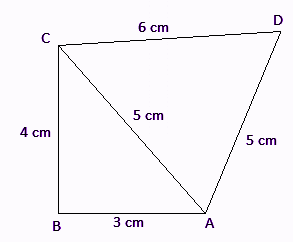Area of quadrilateral ABCD = area of ∆ABC + area of ∆ACD

Since, sides of ∆ABC form a Pythagorian triplet.

Area of ∆ABC = 1/2 x base x altitude

= 1/2 × AB × BC

= 1/2 x 3 x 4

= 6 cm²

∆ACD is an isosceles triangle with equal side (a) = 5 cm and third side (b) = 6 cm.

Area of an isosceles ∆ACD

= b/4 x √(4a2 – b2)

= 6/4 x √[4(5)2 – 62]

= 6/4 x √[4(25) – 36]

= 6/4 x √(100 – 36)

= 6/4 x √64

= 6/4 x 8

= 12 cm2

Area of quadrilateral = 6 + 12 = 18 cm2

Question 11: Find the area of the quadrilateral whose sides are 9 cm, 40 cm, 28 cm and 15 cm and angle between the first two sides is a right angle.

Solution:

Let ABCD is a quadrilateral in which AB = 9 cm, BC = 40 cm, CD = 28 cm and DA = 15 cm.

Angle between AB and BC is the right angle.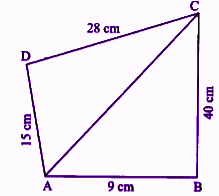In right triangle ABC,

AC = √(92 + 402) = √(81 + 1600) = √1681 = 41 cm

Area of triangle ABC = 1/2 × 9 × 40 = 180 cm2

In triangle ACD,

a = 15 cm, b = 28 cm, c = 41 cm

Semi-perimeter = s = (a + b + c)/2 = (15 + 28 + 41)/2 = 84/2 = 42 cm

Area of triangle ACD = √[s(s – a)(s – b)(s – c)]

= √[42 (42 – 15)(42 – 28)(42 – 41)]

= √(42 × 27 × 14 × 1)

=√(3 × 2 × 7 × 3 × 3 × 3 × 7 × 2)

= 3 × 3 × 2 × 7

= 126 cm2

Area of quadrilateral = Area of triangle ABC + Area of triangle ACD

= 180 + 126

= 306 cm2

Question 12: Find the area of a parallelogram whose two adjacent sides are 50 cm and 40 cm, respectively and diagonal is 30 cm.

Solution:

Given,

Lengths of two adjacent sides are 50 cm and 40 cm.

Diagonal = 30 cm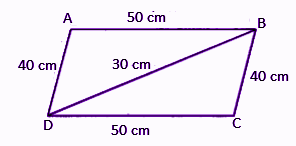In triangle ABD,

a = 40 cm, b = 50 cm, c = 30 cm

Semi-perimeter = s = (a + b + c)/2 = (40 + 50 + 30)/2 = 120/2 = 60 cm

Area of triangle ABD = √[s(s – a)(s – b)(s – c)]

= √[60 (60 – 40)(60 – 50)(60 – 30)]

= √(60 × 20 × 10 × 30)

=√(3 × 2 × 2 × 5 × 2 × 2 × 5 × 5 × 2 × 5 × 3 × 2)

= 3 × 2 × 2 × 2 × 5 × 5

= 600 cm2

Area of parallelogram = 2 × area of triangle ABD

= 2 × 600

= 1200 m2

Question 13: Find the area of a parallelogram whose diagonal is 5.2 cm and the length of each perpendicular from the opposite vertices to the diagonal is 3.5 cm.

Solution:

Given,

Length of offsets of the parallelogram is 3.5 cm.

Diagonal = 5.2 cm

Area of parallelogram = ½ × diagonal × (sum of offsets)

= ½ × 5.2 × (3.5 + 3.5)

= 2.6 × 7

= 18.2 cm2

Question 14: A plot of land has a shape of a parallelogram. It is to be covered by mud. Find the cost of spreading mud at the rate of Rs. 100 per square metre while the adjacent sides of the plot are 39 m and 25 m and the diagonal is 56 m.

Solution:

Given,

Adjacent sides of a parallelogram shaped plot measures 39 m and 25 m.

Diagonal = 56 m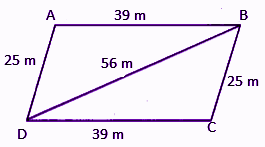In triangle ABD,

a = 25 m, b = 39 m, c = 56 m

Semi-perimeter = s = (a + b + c)/2 = (25 + 39 + 56)/2 = 120/2 = 60 cm

Area of triangle ABD = √[s(s – a)(s – b)(s – c)]

= √[60 (60 – 25)(60 – 39)(60 – 56)]

= √(60 × 35 × 21 × 4)

=√(3 × 2 × 2 × 5 × 7 × 5 × 7 × 3 × 2 × 2)

= 3 × 2 × 2 × 5 × 7

= 420 sq. m

Area of parallelogram = 2 × area of triangle ABD

= 2 × 420

= 840 sq. m

Cost of spreading mud for 1 sq. m = Rs. 100

Total cost of spreading mud = Rs. 100 × 840

= Rs. 84000

## RBSE Maths Chapter 11: Exercise 11.3 Textbook Important Questions and Solutions

Question 15: Sides of a cyclic quadrilateral shaped ground are 72 m, 154 m, 80 m and 150 m, respectively. Find its area. Find the total expenditure of the cost of tiling is Rs 5 per square metre.

Solution:

Let the sides of a cyclic quadrilateral be:

a = 72 m, b = 154 m, c = 80 m, d = 150 m

Semi-perimeter = s = (a + b + c + d)/2 = (72 + 154 + 80 + 150)/2

= 456/2 = 228 m

Area of the ground of cyclic quadrilateral shape = √[(s – a)(s – b)(s – c)(s – d)]

= √[(228 – 72)(228 – 154)(228 – 80)(228 – 150)]

= √(156 × 74 × 148 × 78)

=√(78 × 2 × 74 × 74 × 2 × 78)

= 78 × 74 × 2

= 11544 sq. m

Cost of paving the tiles on the ground 1 sq. m is Rs 5.

∴ Cost of paving the tiles on the ground 11,544 sq. m = 11,544 x 5 = Rs 57,720

Question 16: Diagonals of a rhombus are 25 cm and 42 cm. Find its area and perimeter.

Solution:

Given,

Length of diagonals are 25 cm and 42 cm.

i.e. d1 = 25 cm, d2 = 42 cm

Area of a rhombus

= 1/2 x product of its diagonals

= 1/2 x 25 x 42

= 25 x 21

= 525 cm2

Measure of a side = 1/2 × √(d12 + d22)

= 1/2 × √[(25)2 + (42)2]

= 1/2 × √(625 + 1764)

= 1/2 × √2389

= 1/2 × 48.87

= 24.435 cm

Perimeter of rhombus = 4 × side

= 4 × 24.435

= 97.74 cm

Question 17: Perimeter of a rhombus is 40 m and the length of its diagonal is 12 m, then find its area.

Solution:

Given,

Perimeter of rhombus = 4 × side

40 = 4 × side

Side = 40/4 = 10 m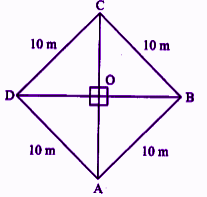Let one of its diagonal be BD.

i. e. BD = 12 m

∴ OB = OD = 6 m

In right ∆OCD,

OC² + OD² = CD²

OC² = CD² – OD²

OC² = (10)² – (6)²

OC² = 100 – 36

OC = √64 = 8 m

∴ Other diagonal AC = 2 x OC

= 2 x 8 = 16 m

i.e. d1 = 12 m, d2 = 16 m

And area of rhombus ABCD = 1/2 × d1 × d2

= 1/2 × 12 × 16

= 96 m2

Question 18: A trapezium shaped field whose parallel sides are 42 m and 30 m and the other sides are 18 m and 18 m. Find its area.

Solution:

Let ABCD be a trapezium in which AB = 42 m and CD = 30 m, BC = DA = 18 m.

Draw CE ∥ DA and CF ⊥ AB.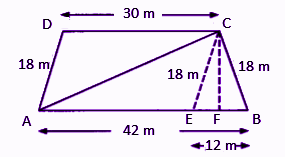In triangle BCE,

Let a = 18 m and b =12 m

Area of ΔBCE = b/4 √(4a2 – b2)

= 12/4 √[4(18)2 – 122]

= 3 √(4 × 324 – 144)

= 3 √(1296 – 144)

= 3 √1152

= 3 × 33.94

= 101.82 m2

Now, area of triangle BCE = 1/2 × BE × CF

101.82 = 1/2 × 12 × CF

CF = 101.82/6

CF = 16.9 m

Area of parallelogram AECD = AE × CF

= 30 × 16.9

= 507 m2

Area of trapezium ABCD = Area of parallelogram AECD + Area of triangle BCE

= 507 + 101.82

= 608.82 m2

Question 19: If the area of a trapezium is 350 cm2 and lengths of its parallel sides are 26 cm and 44 cm, then find the distance between the parallel sides.

Solution:

Given,

Lengths of parallel sides of a trapezium are 26 cm and 44 cm.

Let h cm be the distance between two parallel sides.

Area of trapezium = 350 cm2 (given)

1/2 × (Sum of parallel sides) × Distance between the parallel sides = 350

1/2 × (26 + 44) × h = 350

70h = 350 × 2

h = 700/70

h = 10 cm

Therefore, the distance between the parallel sides of the trapezium = 10 cm

Question 20: A table has a shape of a trapezium. The parallel sides of the table are 8 m and 16 m. If the area of the table is 108 m2, then find the width (distance between parallel sides) of the table.

Solution:

Given,

Lengths of parallel sides of a trapezium shaped table are 8 m and 16 m.

Let h m be the width (distance between two parallel sides) of a table.

Area of table (trapezium) = 108 m2 (given)

1/2 × (Sum of parallel sides) × Distance between the parallel sides = 108

1/2 × (8 + 16) × h = 108

24h = 108 × 2

h = 216/24

h = 9 m

Therefore, the width of the table (distance between the parallel sides of the trapezium) = 9 m

## RBSE Maths Chapter 11: Exercise 11.4 Textbook Important Questions and Solutions

Question 21: Find the area and perimeter of rectangles of following measure:

(i) length = 9.5 m, breadth = 7.5 m

(ii) length = 125 m, breadth = 75 m

(iii) length = 12.5 cm, breadth = 7.5 cm

Solution:

Perimeter of rectangle = 2(Length + Breadth)

Area of rectangle = Length × Breadth

(i) Length = 9.5 m, Breadth = 7.5 m

Perimeter of a rectangle

= 2(9.5 + 7.5)

= 2 x 17

= 34 m

Area of rectangle = 9.5 x 7.5 = 71.25 m²

(ii) Length = 125 m, Breadth = 75 m

Perimeter of a rectangle

= 2(125 + 75)

= 400 m

Area of rectangle = 125 x 75 = 9375 m²

(iii) Length = 12.5 cm, Breadth = 7.5 cm

Perimeter of a rectangle

= 2(12.5 + 7.5)

= 2 x 20

= 40 cm

Area of rectangle = 12.5 x 7.5 = 93.75 cm²

Question 22: Find the area and perimeter of the square of a side 5.3 m.

Solution:

Given,

Side of the square = 5.3 m

Perimeter of square = 4 x side

= 4 x 5.3

= 21.2 m

Area of square = side x side

= 5.3 x 5.3

= 28.09 m2

Question 23: After running 4 rounds to a square field, a distance covered is 16 km. Find the length and area of the ground.

Solution:

Given,

Distance covered in 4 rounds = 16 km

∴ Distance covered in 1 round = 16/4 = 4 km = 4000 m

Hence, perimeter of the ground = 4000 m

4 x side = 4000 m

Side = 1000 m

Area of the square field = 1000² = 1000000 m²

Therefore, the length of the square field is 1000 m and area is 1000000 m2.

Question 24: A rectangular field is 75 m long and 45 m wide. How many beds of 5 m long and 3 m wide can be made in it?

Solution:

Given,

Length of the rectangular field = 75 m

Width of the rectangular field = 45 m

Area of rectangular field

= length x width

= 75 × 45 m2

Area of one flower-bed

= 5 × 3

= 15 m²

∴ Number of beds = Area of rectangular field/ Area of one bed

= (75 × 45)/15

= 225

Question 25: The length of a room is 10 m and breadth is 5 m. How many square pieces of area 50 cm2 are required on its floor? Find the expenditure of paving the square pieces on the floor at the rate of Rs. 20 per piece.

Solution:

Area of room = length x breadth

= 10 × 5

= 50 m2

Area of one square = 50 cm2

= 50/ (100 × 100) m2

Required number of squares = Area of room/Area of one square

= 50/ [50/(100 × 100)]

= 100 × 100

= 10000

Cost of paving one square = Rs. 20 (given)

Cost of paving 10000 squares = Rs. 20 × 10000

= Rs. 200000

Question 26: Area of a square ground is 2025 m2. There is a 3.5 m wide path outside around it then find the area of the path.

Solution: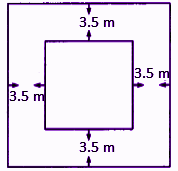Given,

Area of square ground = 2025 m2

Side of the square ground = √Area = √2025 = 45 m

The length of the side of the ground including the path = 45 + (3.5 + 3.5) = 52 m

∴ Area of the ground including the path = 52 × 52 = 2704 m²

∴ Area of the path = Area of the ground including path – Area of the square ground

= 2704 – 2025

= 679 m²

Question 27: A rectangular room is 40 m long and 25 m wide. There is a road 2.5 m wide around it. Tiles of size 50 cm × 70 cm are to be paved on it. Find the number of pieces.

Solution:

Area of a rectangular room

= 40 x 25 = 1000 m²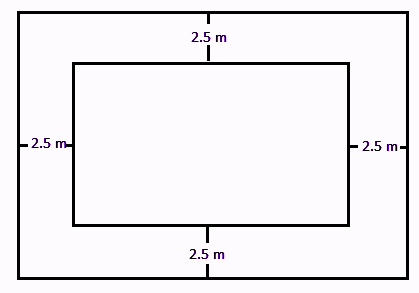Area of rectangular room with road

= (40 + 2.5 + 2.5) × (25 + 2.5 + 2.5)

= 45 × 30

= 1350 m²

Area of road = outer area – inner area

= 1350 – 1000 = 350 m²

Area of one tile = 50 × 70 = 3500 cm2 = 0.35 m2

Required number of tiles = Area of the road/ Area of one tile

= 350/0.35

= 35000/35

= 1000

Question 28: A rectangular ground is 60 m long and 40 m wide. There is a 4 m wide path parallel to its length and width inside the ground. Find the expenditure of spreading mud on the path at the rate of Rs. 100 per square metre.

Solution:

Given,

Length of the rectangular ground = 60 m

Width of the rectangular ground = 40 m

Width of the path = 4 m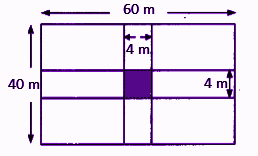Area of the path along length

= 60 × 4 = 240 m²

Area of the path along width

= 40 × 4 = 160 m²

Common area = 4 × 4 = 16 m²

∴ Total area of the path

= 240 + 160 – 16

= 384 m²

Expenditure of spreading mud per m² = Rs 100 (given)

∴ Total expenditure of spreading mud on 384 m² = 384 × Rs. 100 = Rs. 38,400

Question 29: Find the area and perimeter of the given diagram.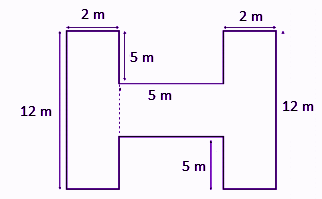Solution:

In the given figure, mark the blocks P, Q and R as shown below.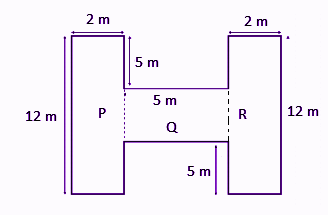Area of block P = 12 × 2 = 24 m²

Area of block Q = 5 × 2 = 10 m²

Area of block R = 12 × 2 = 24 m²

∴ Total area of the given figure = 24 + 10 + 24 = 58 m²

Perimeter of the whole figure

= 2 + 12 + 2 + 5 + 5 + 5 + 2 + 12 + 2 + 5 + 5 + 5

= 62 m

Question 30: The length, breadth and height of a room are respectively 15.35 m, 4.65 m and 6.50 m. If there are 4 doors of size 1.5 m × 1.3 m and 3 windows of size 1.5 m × 1.2 m, then find the expenditure on whitewashing the room at the rate of Rs. 4.30 per square metre.

Solution:

According to the given,

l = 15.35 m, b = 4.65 m and h = 6.50 m

Area of four walls

= 2 x (l + b) × h

= 2 x (15.35 + 4.65) × 6.50

= 2 × 20 × 6.50

= 260 m²

Area of four doors

= 4 × 1.5 × 1.3

= 7.8 m²

Area of 3 windows

= 3 × 1.5 × 1.2

= 5.4 m²

The area of the four walls excluding door and windows

= 260 – (7.8 + 5.4)

= 260 – 13.2

= 246.8 m²

Cost of whitewashing 1 m² = Rs. 4.30 (given)

Cost of whitewashing 246.8 m²

= 246.8 × Rs. 4.30

= Rs. 1061.24

Question 31: A water tank is 10 m long, 8 m wide and 2 m deep. Find the expenditure of repairing its four walls and floor at the rate of Rs. 15 per square metre.

Solution:

Given,

Length = l = 10 m

Breadth = b = 8 m

Deep = h = 2 m

Floor area of water tank = length x breadth = 10 x 8 = 80 m²

Area of four walls of the water tank

= 2(l + b) x h

= 2 x (10 + 8) x 2

= 2 x 18 x 2

= 72 m2

Total area of the water tank = 72 + 80 = 152 m²

Cost of repairing 1 m² = Rs. 15 (given)

∴Cost of repairing 152 m² = Rs. 15 x 152 = Rs. 2280

### RBSE Maths Chapter 11: Additional Important Questions and Solutions

Question 1: If the side of an equilateral triangle is 8 cm, then area of the triangle will be:

(A) 16√3 cm2

(B) 8√3 cm2

(C) 64√3 cm2

(D) 4√3 cm2

Solution:

Given,

Side of an equilateral triangle = a = 8 cm

Area = (√3)/4 a2

= (√3)/4 × 8 × 8

= 16√3 cm2

Question 2: The sides of a triangle are 40 cm, 70 cm and 90 cm, area of triangle will be:

(A) 600√5 cm2

(B) 500√6 cm2

(C) 482√5 cm2

(D) 60√5 cm2

Solution:

Let a, b and c be the sides of the triangle.

a = 40 cm, b = 70 cm and c = 90 cm

Semi-perimeter of triangle:

s = (a + b + c)/2

= (40 + 70 + 90)/2

= 200/2

= 100

Area of triangle = √[s(s – a)(s – b)(s – c)]

= √[100 (100 – 40)(100 – 70)(100 – 90)]

= √(100 × 60 × 30 × 10)

=√( 10 × 10 × 5 × 2 × 2 × 3 × 3 × 10 × 10)

= 10 × 10 × 2 × 3 √5

= =600√5 cm2

Question 3: Each equal side of an isosceles triangle is 6 cm and the other side is 8 cm, then area of triangle will be:

(A) 8√5 cm2

(B) 5√8 cm2

(C) 3√55 cm2

(D) 3√8 cm2

Solution:

Let a = 6 cm and b = 8 cm

Area of an isosceles triangle = (b/4) × √(4a2 – b2)

= (8/4) × √[4(6)2 – 82]

= 2 √[4(36) – 64]

= 2 √(144 – 64)

= 2 √80

= 2 √(4 × 4 × 5)

= 2 × 4√5

= 8√5 cm2

Question 4: The perimeter of an equilateral triangle is 60 cm, then its area will be:

(A) 400√3 cm2

(B) 100√3 cm2

(C) 50√3 cm2

(D) 200√3 cm2

Solution:

Given,

Perimeter of an equilateral triangle = 60 cm

3a = 60

a = 60/3 = 20 cm

Area of an equilateral triangle = (√3)/4 a2

= (√3)/4 × 20 × 20

= 100√3 cm2

Question 5: Area of a right-triangle is 36 cm2 and its base is 9 cm, then the length of perpendicular will be:

(A) 8 cm

(B) 4 cm

(C) 16 cm

(D) 32 cm

Solution:

Area of a right triangle = ½ × base × height

36 = ½ × 9 × height

Height = (36 × 2)/9 = 8

Therefore, the length of the perpendicular = 8 cm

Question 6: If the side of a square is 10 cm, then its perimeter will be:

(A) 20 cm

(B) 10 cm

(C) 40 cm

(D) 30 cm

Solution:

Given,

Side of a square = 10 cm

Perimeter of square = 4 × side

= 4 × 10

= 40 cm

Question 7: If the diagonals of a rhombus are 8 cm and 6 cm, then its area will be:

(A) 48 cm2

(B) 24 cm2

(C) 12 cm2

(D) 96 cm2

Solution:

Given,

Lengths of the diagonals are 8 cm and 6 cm.

Area of rhombus = ½ × Product of two diagonals

= ½ × 8 × 6

= 48/2

= 24 cm2

Question 8: If the perimeter of room is 40 m and height is 4 m, then area of its four walls will be:

(A) 40 m2

(B) 80 m2

(C) 120 m2

(D) 160 m2

Solution:

Given,

Perimeter of room = 40 m

Area of 4 walls = Perimeter × Height

= 40 × 4

= 160m2

Question 9: What will be the length of the side of an equilateral triangle whose area is 9√3 cm2?

Solution:

Area of an equilateral triangle = (√3)/4 × (side)2

9√3 =(√3)/4 × (side)2

36 = (side)2

side = √36 = 6 cm

Therefore, length of the side = 6 cm

Question 10: Write the formula to find the area of cyclic quadrilateral.

Solution:

Area of cyclic quadrilateral = √[(s – a)(s – b)(s – c)(s – d)]

Where:

a, b, c, d are the measures of sides of cyclic quadrilateral

s = Semi-perimeter of the cyclic quadrilateral = (a + b + c + d)/2

Question 11: Area of a square is 144 m2 Are, then write its perimeter.

Solution:

Given,

Area of square =144 m2 Are

(side)2 = 144 × 100 m2 (∵ 1 Are = 100 m2)

(side)2 = 14400 m2

⇒ side = √14400 = 120 m

Therefore, Perimeter = 4 × side = 4 × 120 = 480 m

Question 12: If the base of a parallelogram is 18 m and area is 174.60 m2, then write its height.

Solution:

Given,

Base of the parallelogram = 18 m

Area of parallelogram = 174.60 m2

Base × Height = 174.60

18 × Height = 174.60

Height = 174.60/18 = 9.7 m

Question 13: Write the area of a quadrilateral whose diagonal is 6 cm and the sum of internal perpendiculars is 12 cm.

Solution:

Given,

Diagonal = 6 cm

Sum of the length of perpendiculars (offsets) is 12 cm.

Area of quadrilateral = ½ × (sum of offsets) × diagonal

= ½ × 12 × 6

= 3 × 12

= 36 cm2

Question 14: Area of an isosceles triangle is 12 cm2. Find its base, if the length of its equal sides is 5 cm.

Solution:

Given,

Area of an isosceles triangle = 12 cm2

Length of the equal sides = a = 5 cm

Area of an isosceles triangle = b/4 √(4a2 – b2)

12 = b/4 √[4(5)2 – b2]

12 × 4 = b√(4 × 25 – b2)

48 = b√(100 – b2)

Squaring on both sides,

2304 = b2(100 – b2)

2304 = 100b2 – b4

b4 – 100b2 + 2304 = 0

b4 – 64b2 – 36b2 + 2304 = 0

b2(b2 – 64) – 36(b2 – 64) = 0

(b2 – 36)(b2 – 64) = 0

b2 – 36 = 0 or b2 – 64 = 0

b2 = 36 or b2 = 64

b = 6 or b = 8

Therefore, the base of the triangle is 8 cm or 6 cm.